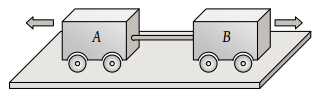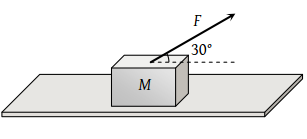The maximum speed that can be achieved without skidding by a car on a circular unbanked road of radius R and coefficient of static friction μ, is

(1) $\mu Rg$

(2) $Rg\sqrt{\mu }$

(3) $\mu \sqrt{Rg}$

(4) $\sqrt{\mu Rg}$

Concept Questions :-

Friction
High Yielding Test Series + Question Bank - NEET 2020

Difficulty Level:

A car is moving along a straight horizontal road with a speed v0. If the coefficient of friction between the tyres and the road is μ, the shortest distance in which the car can be stopped is

(1) $\frac{{v}_{0}^{2}}{2\mu g}$

(2) $\frac{{v}_{0}}{\mu g}$

(3) ${\left(\frac{{v}_{0}}{\mu g}\right)}^{2}$

(4) $\frac{{v}_{0}}{\mu }$

Concept Questions :-

Friction
High Yielding Test Series + Question Bank - NEET 2020

Difficulty Level:

Two carts of masses 200 kg and 300 kg on horizontal rails are pushed apart. Suppose the coefficient of friction between the carts and the rails are same. If the 200 kg cart travels a distance of 36 m and stops, then the distance travelled by the cart weighing 300 kg is(1) 32 m

(2) 24 m

(3) 16 m

(4) 12 m

Concept Questions :-

Friction
High Yielding Test Series + Question Bank - NEET 2020

Difficulty Level:

A block of mass 50 kg can slide on a rough horizontal surface. The coefficient of friction between the block and the surface is 0.6. The least force of pull acting at an angle of 30° to the upward drawn vertical which causes the block to just slide is

(1) 29.43 N

(2) 219.6 N

(3) 21.96 N

(4) 294.3 N

Concept Questions :-

Friction
High Yielding Test Series + Question Bank - NEET 2020

Difficulty Level:

Assuming the coefficient of friction between the road and tyres of a car to be 0.5, the maximum speed with which the car can move round a curve of 40.0 m radius without slipping, if the road is unbanked, should be

(1) 25 m/s

(2) 19 m/s

(3) 14 m/s

(4) 11 m/s

Concept Questions :-

Friction
High Yielding Test Series + Question Bank - NEET 2020

Difficulty Level:

Consider a car moving along a straight horizontal road with a speed of 72 km/h. If the coefficient of kinetic friction between the tyres and the road is 0.5, the shortest distance in which the car can be stopped is (g = 10 m/s2

(1) 30 m

(2) 40 m

(3) 72 m

(4) 20 m

Concept Questions :-

Friction
High Yielding Test Series + Question Bank - NEET 2020

Difficulty Level:

On the horizontal surface of a truck (μ = 0.6), a block of mass 1 kg is placed. If the truck is accelerating at the rate of 5m/sec2 then frictional force on the block will be

(1) 5 N

(2) 6 N

(3) 5.88 N

(4) 8 N

Concept Questions :-

Pseudo force
High Yielding Test Series + Question Bank - NEET 2020

Difficulty Level:

A vehicle of mass m is moving on a rough horizontal road with momentum P. If the coefficient of friction between the tyres and the road be μ, then the stopping distance is

(1) $\frac{P}{2\mu \text{\hspace{0.17em}}m\text{\hspace{0.17em}}g}$

(2) $\frac{{P}^{2}}{2\mu \text{\hspace{0.17em}}m\text{\hspace{0.17em}}g}$

(3) $\frac{P}{2\mu \text{\hspace{0.17em}}m{\text{\hspace{0.17em}}}^{2}g}$

(4) $\frac{{P}^{2}}{2\mu \text{\hspace{0.17em}}m{\text{\hspace{0.17em}}}^{2}g}$

Concept Questions :-

Friction
High Yielding Test Series + Question Bank - NEET 2020

Difficulty Level:

A block of mass M = 5 kg is resting on a rough horizontal surface for which the coefficient of friction is 0.2. When a force F = 40 N is applied, the acceleration of the block will be (g = 10 m/s2)(1) 5.73 m/sec2

(2) 8.0 m/sec2

(3) 3.17 m/sec2

(4) 10.0 m/$se{c}^{2}$

Concept Questions :-

Friction
High Yielding Test Series + Question Bank - NEET 2020

Difficulty Level:

Consider a car moving on a straight road with a speed of 100 m/s. The distance at which car can be stopped is $\left[{\mu }_{k}=0.5\right]$

(1) 100 m

(2) 400 m

(3) 800 m

(4) 1000 m

Concept Questions :-

Friction